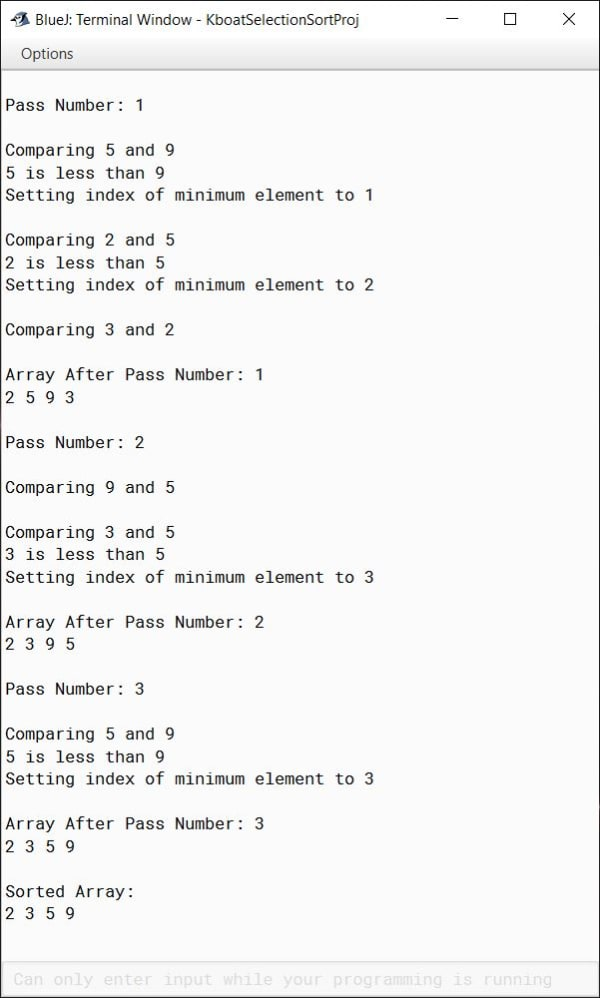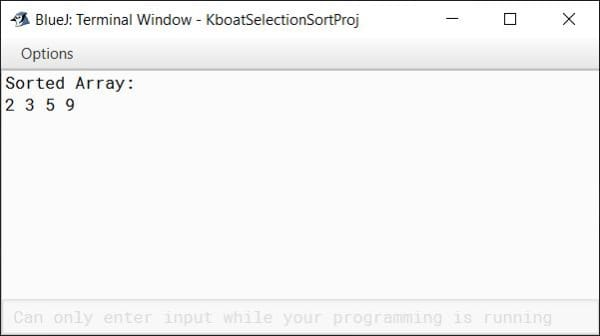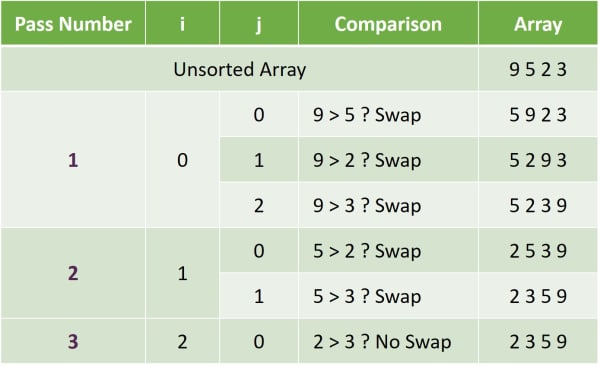# Selection Sort

## ICSE Computer Applications

Let’s take the same cards we used for Bubble Sort and try sorting them using the Selection Sort method.

Like in Bubble Sort, here also we will make multiple passes through the cards to sort them. In the first pass, we will look at all the cards and find the smallest card. Two is the smallest of these four cards. We will swap this two with the first card. Now card two is in its correct position. So, after the first pass we have sorted one card. In the next pass, we will find the smallest of these 3 cards. It is three. We will swap it with the second card. At the end of second pass, the first two cards are in their correct position. In the third pass, we will find the smallest of these 2 cards which is five and swap it with this third card nine. At the end of this pass we have sorted all the 4 cards.

To summarize this process, we can say that Selection Sort divides the array into two parts — sorted sub-array and unsorted sub-array. In each pass, it finds the minimum element of the unsorted sub-array and swaps it with the leftmost unsorted element moving the sorted sub-array one element to the right.

## Selection Sort Java Program

```public class KboatSelectionSort
{
public static void displayArray(int arr[]) {
for (int i = 0; i < arr.length; i++) {
System.out.print(arr[i] + " ");
}
System.out.println();
}

public static void main(String args[]) {
int arr[] = {9, 5, 2, 3};
int n = arr.length;

//Selection Sort
for (int i = 0; i < n - 1; i++) {
System.out.println("\nPass Number: " + (i + 1));
int idx = i;
for (int j = i + 1; j < n; j++) {
System.out.println("\nComparing "+ arr[j]
+ " and " + arr[idx]);
if (arr[j] < arr[idx]) {
System.out.println(arr[j]
+ " is less than "
+ arr[idx]);
System.out.println("Setting index of minimum element to "
+ j);
idx = j;
}
}

int t = arr[i];
arr[i] = arr[idx];
arr[idx] = t;

System.out.println("\nArray After Pass Number: " + (i + 1));
displayArray(arr);
}

System.out.println("\nSorted Array:");
displayArray(arr);
}
}```

#### OutputLike Bubble Sort program, here too I have added this `displayArray` method and a few additional print statements to get a detailed output explaining each step of Selection Sort process. After going over the program, I will remove these print statements and show you the program to give just the sorted array as the output.

```int arr[] = {9, 5, 2, 3};
int n = arr.length;```

We start the main method by declaring and initializing the array `arr` and storing its size in int variable `n`. Notice that it is the same array that we used for Bubble Sort.

```for (int i = 0; i < n - 1; i++) {
System.out.println("\nPass Number: " + (i + 1));
int idx = i;
for (int j = i + 1; j < n; j++) {
System.out.println("\nComparing "+ arr[j]
+ " and " + arr[idx]);
if (arr[j] < arr[idx]) {
System.out.println(arr[j]
+ " is less than "
+ arr[idx]);
System.out.println("Setting index of minimum element to "
+ j);
idx = j;
}
}

int t = arr[i];
arr[i] = arr[idx];
arr[idx] = t;

System.out.println("\nArray After Pass Number: " + (i + 1));
displayArray(arr);
}```

After this the core Selection Sort logic starts. The outer loop controls the number of passes we make through the array. To sort an array of size n, we need to make n – 1 passes through it. Size of `arr` is 4 so it will take at the most 3 passes to sort it completely. So, the outer loop runs from 0 which is the starting index of the array and keeps iterating as long as `i` is less than `n – 1`. We print the pass number and then we declare an int variable `idx` and initialize it with `i`. This variable `idx` will hold the index of the smallest element in the unsorted part of the array. Initializing it with `i` means that we start the pass by assuming that the first element of the unsorted part of the array is the smallest element. Then as we will look over other elements in the inner loop, we will update the value of `idx` if we find an element which is smaller than the element at `idx`.

In the inner loop, we go over each element of the unsorted part of the array and check if it is smaller than the smallest element that we have found so far. Here, `j` is initialized to `i + 1`. The reason for this is, in each pass, the length of the sorted part increases by 1. Recall from the cards example that as we were making our passes through the cards, they were getting sorted from left to right. So, we compare the cards from `i + 1` till the end of the array using the condition `j < n`. If the if check — `if (arr[j] < arr[idx])` is true, it means that `arr[j]` is the smallest element we have seen so far, and we update `idx` accordingly.

At the end of the inner loop, we have the index of the smallest element in `idx`. We swap the element at index `idx` with the element at index `i`. Then we print the array just before the outer loops ends.

```System.out.println("\nSorted Array:");
displayArray(arr);```

Once these nested loops complete executing, our array is sorted, and we display the final sorted array as the output of the program. And with this, our Selection Sort program completes.

Don’t worry if you are still feeling a bit uncomfortable with Selection Sort. Next, we will do a dry run of the program which will make its working clear.

The int variable `n` gets the value of 4 as there are 4 elements in `arr`. The outer for loop starts by initializing `i` to 0. At this point the entire array is unsorted so the size of our sorted sub-array is 0. Condition of outer loop is true, so we start our first pass through the array. `idx` gets the value of 0. Remember, `idx` will hold the index of the smallest element of the unsorted sub-array. After that the inner loop starts and `j` is initialized to 1. `arr[j]` is five, `arr[idx]` is nine, the two are compared and as five is less than nine, we update the index of the minimum element to 1. Program control goes back to the beginning of inner for loop to compare next element. `j` becomes 2, condition of inner for is true. `arr[j]` is now two and `arr[idx]` is five, the two are compared and as two is less than five, we again update the index of the minimum element to 2. Next iteration of inner for loop starts, `j` becomes 3, condition is true so `arr` which is 3 is compared to `arr` which is 2. `idx` is not updated as 3 is greater than 2 so the condition is false. We don’t want to update `idx` this time as it is already pointing to the smallest element. Program control goes back to the beginning of inner for loop. `j` is incremented to 4. This time the condition is false so program control jumps to the statement after the inner for. The next three statements swap the values of 9 and 2. Next, we print the updated array after the swap. With this, our first pass through the array completes and we have sorted the first element of the array. Length of the sorted sub-array is now 1.

Program control goes back to the beginning of the outer for. `i` becomes 1. Condition is true, we start our second pass through the array. This time `idx` is set to 1, the index of first element of our unsorted sub-array. Inner loop starts, `j` becomes 2. Condition is true. `arr[j]` which nine is compared to `arr[idx]` which is five. The if condition is false so `idx` is not updated. In the next iteration of inner for, `j` becomes 3, condition is true. `arr[j]` is three, `arr[idx]` is five like before. The if condition becomes true and `idx` is updated to 3. Program control goes back to the beginning of inner for and increments `j` to 4. Loop condition becomes false and program control comes to this statement after the inner for. 5 and 3 are swapped. Its first two elements are sorted. Length of the sorted sub-array is now 2.

Program control again goes back to the beginning of the outer for. `i` becomes 2. Loop condition is true, we start our third pass through the array. `idx` is set to 2. Inner for loop begins and `j` is initialized to 3. Condition is true. `arr[j]` is five and `arr[idx]` is nine. Both are compared and the condition is found true. `idx` is updated to 3. Program control goes back to the beginning of inner for, `j` is incremented to 4. Condition becomes false. Inner for loop is not executed and program control comes here. 9 and 5 are swapped. These two statements again print the updated array to the console.

Program control goes back to the beginning of outer for loop and increments `i` to 3. This time the condition is false. Outer loop stops executing. Program control comes to the statement after the outer for loop. The sorted array is printed to the console and our program completes.

Now that we have the complete understanding of Selection Sort program, lets remove all the extra print statements and the `displayArray` method we added for this detailed output and just look at the Selection Sort program only.

```public class KboatSelectionSort
{
public static void main(String args[]) {
int arr[] = {9, 5, 2, 3};
int n = arr.length;

//Selection Sort
for (int i = 0; i < n - 1; i++) {
int idx = i;
for (int j = i + 1; j < n; j++) {
if (arr[j] < arr[idx])
idx = j;
}

int t = arr[i];
arr[i] = arr[idx];
arr[idx] = t;
}

System.out.println("Sorted Array:");
for (int i = 0; i < n; i++) {
System.out.print(arr[i] + " ");
}
}
}```

#### OutputThis table summarizes the Selection Sort steps of sorting the array arr which we discussed just now. You can use this as a reference.## Bubble Sort v/s Selection Sort

Let’s conclude our discussion of sorting by comparing Bubble Sort and Selection Sort.

1. Both Bubble Sort and Selection Sort need the same number of passes through the array to sort it completely.
2. Bubble Sort compares adjacent elements and swaps them if they are in wrong order. Selection Sort selects the smallest element from unsorted sub-array and swaps it with the leftmost unsorted element.
3. Selection Sort makes fewer swaps compared to Bubble Sort which makes it a little bit faster.
4. In Bubble Sort, array is sorted from last element to first element whereas in Selection Sort, array is sorted from first element to the last element.